## How to Calculate and Solve for Shear Modulus | Mechanical Properties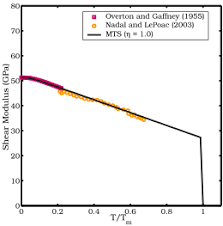The image above represents shear modulus.

To compute for shear modulus, two essential parameters are needed and these parameters are Shear Stress (τ) and Shear Strain (γ).

The formula for calculating shear modulus:

G = τ/γ

Where:

G = Shear Modulus
τ = Shear Stress
γ = Shear Strain

Given an example;
Find the shear modulus when the shear stress is 15 and the shear strain is 3.

This implies that;

τ = Shear Stress = 15
γ = Shear Strain = 3

G = τ/γ
G = 15/3
G = 5

Therefore, the shear modulus is 5 Pa.

Calculating the Shear Stress when the Shear Modulus and the Shear Strain is Given.

τ = G x γ

Where:

τ = Shear Stress
G = Shear Modulus
γ = Shear Strain

Let’s solve an example;
Find the shear stress when the shear modulus is 20 and the shear strain is 4.

This implies that;

G = Shear Modulus = 20
γ = Shear Strain = 4

τ = G x γ
τ = 20 x 4
τ = 80

Therefore, the shear stress is 80 Pa.

## How to Calculate and Solve for Young’s Modulus | Mechanical Properties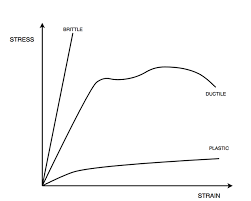The image above represents young’s modulus.

To compute for young’s modulus, two essential parameters are needed and these parameters are Stress and Strain.

The formula for calculating young’s modulus:

Young’s Modulus = Stress / Strain

Given an example;
Find the young’s modulus when the stress is 22 and the strain is 11.

This implies that;

Stress = 22
Strain = 11

Young’s Modulus = Stress / Strain
Young’s Modulus = 22 / 11
Young’s Modulus = 2

Therefore, the young’s modulus is 2 Pa.

## How to Calculate and Solve for Elastic Constant | Hooke’s Law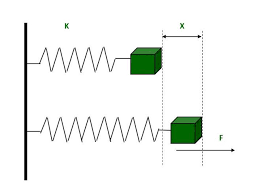The image above represents elastic constant.

To compute for elastic constant, two essential parameters are needed and these parameters are Force (F) and Extension (e).

The formula for calculating elastic constant:

k = F/e

Where:

k = Elastic Constant
F = Force
e = Extension

Given an example;
Find the elastic constant when the force is 30 and the extension is 6.

This implies that;

F = Force = 30
e = Extension = 6

k = F/e
k = 30 / 6
e = 5

Therefore, the elastic constant is 5 Nm-1.

## How to Calculate and Solve for Extension | Hooke’s Law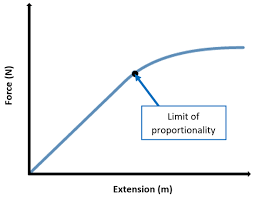The image above represents extension.

To compute for extension, two essential parameters are needed and these parameters are Elastic Constant (k) and Force (F).

The formula for calculating extension:

e = F/k

Where:

e = Extension
F = Force
k = Elastic Constant

Let’s solve an example;
Given that the force is 18 and the elastic constant is 2. Find the extension?

This implies that;

F = Force = 18
k = Elastic Constant = 2

e = F/k
e = 18 / 2
e = 9

Therefore, the extension is 9 m.

## How to Calculate and Solve for Force | Hooke’s Law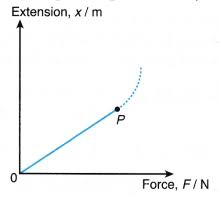The image above represents force.

To compute for force, two essential parameters are needed and these parameters are Elastic Constant (k) and Extension (e).

The formula for calculating force:

F = ke

Where:

F = Force
k = Elastic Constant
e = Extension

Given an example;
Find the force when the elastic constant is 48 and the extension is 6.

The implies that;

k = Elastic Constant = 48
e = Extension = 6

F = ke
F = 48 x 6
F = 288

Therefore, the force is 288 N.

## How to Calculate and Solve for Shear Stress | Mechanical Properties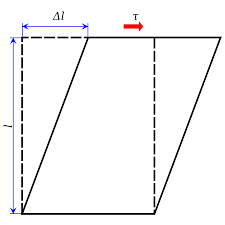The image above represents shear stress.

To compute for shear stress, two essential parameters are needed and these parameters are Shear Force (Fxand Area (A).

The formula for calculating shear stress:

τ = Fx/A

Where:

τ = Shear Stress
Fx = Shear Force
A = Area

Given an example;
Find the shear stress when the shear force is 32 and the area is 4.

This implies that;

Fx = Shear Force = 32
A = Area = 4

τ = Fx/A
τ = 32/4
τ = 8

Therefore, the shear stress is 8 N.

Calculating the Shear Force when the Shear Stress and Area is Given.

Fx = τ x A

Where:

Fx = Shear Force
τ = Shear Stress
A = Area

Let’s solve an example;
Find the shear force when the shear stress is 9 and the area is 4.

This implies that;

τ = Shear Stress = 9
A = Area = 4

Fx = τ x A
Fx = 9 x 4
Fx = 36

Therefore, the shear force is 36 N.

## How to Calculate and Solve for Engineering Strain | Mechanical Properties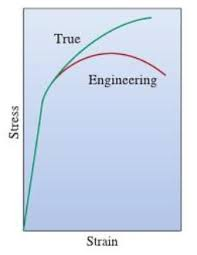The image above represents engineering strain.

To compute for engineering strain, two essential parameters are needed and these parameters are Extension (e) and Length (l).

The formula for calculating engineering strain:

Strain = e/l

Where:

Strain = Engineering Strain
e = Extension
l = Length

Given an example;
Find the engineering strain when the extension is 20 and the length is 5.

This implies that;

e = Extension = 20
l = Length = 5

Strain = e/l
Strain = 20 / 5
Strain = 4

Therefore, the engineering strain is 4.

Calculating the Extension when the Engineering Strain and the Length is Given.

e = Strain x l

Where:

e = Extension
Strain = Engineering Strain
l = Length

Let’s solve an example;
Find the extension when the strain is 2 and the length is 8.

This implies that;

Strain = Engineering Strain = 2
l = Length = 8

e = Strain x l
e = 2 x 8
e = 16

Therefore, the extension is 16.

## How to Calculate and Solve for Engineering Stress | Mechanical Properties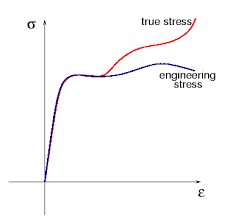The image above represents engineering stress.

To compute for engineering stress, two essential parameters are needed and these parameters are Force (F) and Area (A).

The formula for calculating engineering stress:

Stress = F/A

Where:

F = Force
A = Area

Given an example;
Find the engineering stress when the force is 12 and the area is 4.

This implies that;

F = Force = 12
A = Area = 4

Stress = F/A
Stress = 12 / 4
Stress = 3

Therefore, the engineering stress is 3 Nm-2.

Calculating the Force when the Engineering Stress and the Area is Given.

F = Stress x A

Where:

F = Force
Stress = Engineering Stress
A = Area

Let’s solve an example;
Find the force when the engineering stress is 10 and the area is 2.

This implies that;

Stress = Engineering Stress = 10
A = Area = 2

F = Stress x A
F = 10 x 2
F = 20

Therefore, the force is 20 N.

## How to Calculate and Solve for Initial Grain Diameter | Deformation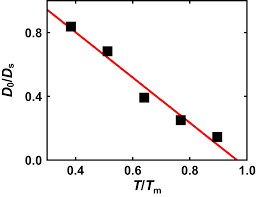The image above represents initial grain diameter.

To compute for initial grain diameter, four essential parameters are needed and these parameters are Average Grain Size Diameter (d), Time (t), Time Independent Constant (k) and Time Independent Constant (n).

The formula for calculating initial grain diameter:

do = (dn – kt)(1/n)

Where:

d = Average Grain Size Diameter
k, n = Time Independent Constants
t = Time

Given an example;
Find the initial grain diameter when the average grain size diameter is 2, the time is 3, the time independent constant is 6 and the time independent constant is 9.

This implies that;

d = Average Grain Size Diameter = 2
k = Time Independent Constant = 6
n = Time Independent Constant = 9
t = Time = 3

do = (dn – kt)(1/n)
do = (29 – (6)(3))(1/9)
do = (512 – (18))(1.992)
do = (494)(1.992)
do = 1.99

Therefore, the initial grain diameter is 1.99 mm.

## How to Calculate and Solve for Strain Hardening Percent of Cold Work | Deformation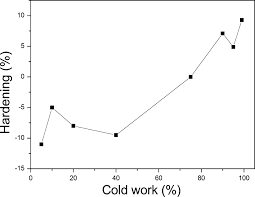The image above represents strain hardening percent of cold work.

To compute for strain hardening percent of cold work, two essential parameters are needed and these parameters are Original Area of Cross Section (Aoand Area after Deformation (Ad).

The formula for calculating strain hardening percent of cold work:

Where:

%CW = Strain Hardening Percent of Cold Work
Ao = Original Area of Cross Section

Given an example;
Find the strain hardening percent of cold work when the original area of cross section is 21 and the area after deformation is 7.

This implies that;

Ao = Original Area of Cross Section = 21
Ad = Area After Deformation = 7